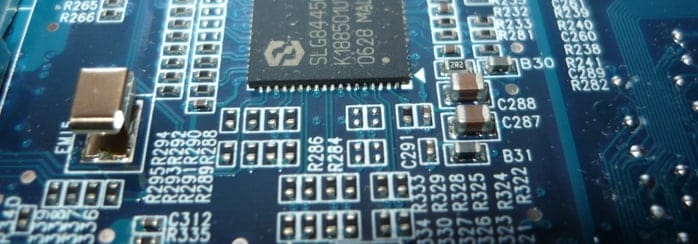# Mesh Current Method and Its ApplicationPreviously, we’ve already introduced the Kirchhoff’s Circuit Law as a separate topic. And today, we shall consider another method for the circuit analysis, which is called the Mesh Current Method. The advantage of this method is that we obtain less unknown variables and equations when we solve the circuit. Let’s consider the T-circuit scheme from the mentioned post and solve it with the help of the Mesh Current Method.Let the first voltage source (or the battery) be $10$ V and the second one – $15$ V. Let the resistances be $R_1 = 15\ \Omega$, $R_2 = 22\ \Omega$ and $R_3 = 30\ \Omega$. Our task is to analyze the circuit and find the currents $I_1$, $I_2$, $I_3$ and the voltage drops on resistances $R_1$, $R_2$, $R_3$.

First, we need to identify the loops in our circuit. As we can see in the scheme above, the first loop consists of the battery $V_1$, and two resistors ($R_2$ and $R_3$). The second one consists of the battery $V_2$, and two resistors ($R_2$ and $R_3$). Let’s choose the clockwise direction of the current in the both loops as shown in the scheme above. Then, we can apply the Kirchhoff’s Voltage Law for both loops (we choose the sign minus for $V_2$ because we travel from positive to negative in the voltage source, thus the potential difference is negative):

$V_1 = I_1(R_1 + R_3) – I_2R_2$ (1)

$V_2 = I_1R_3 – I_2(R_2 + R_3)$ (2)

Finally, after the substitution of the numbers, we get the system of equations for the mesh (or loops) currents:

$10 = 45I_1 – 30I_2$ ,  (3)

$15 = 30I_1 – 52I_2$ . (4)

Then, we can convert this system of equations into the matrix form:

$[V]=[R]\cdot [I]$ (5)

$\begin{bmatrix} 10 \\ 15\end{bmatrix} = \begin{bmatrix} 45 & -30 \\ 30 & -52\end{bmatrix}\cdot\begin{bmatrix} I_1 \\ I_2\end{bmatrix}$

From the Ohm’s law:

$I=R^{-1}V$

And we get:

$[I]=[R^{-1}]\cdot [V]$

here $[R^{-1}]$ is the inverse matrix of $[R]$.

Let’s now find $[R^{-1}]$. For the $2\times 2$ matrix, there is the simple formula to find the inverse matrix:

$\begin{bmatrix} a & b \\ c & d \end{bmatrix}^{-1} = \dfrac{1}{ad-bc}\cdot \begin{bmatrix} d & -b \\ -c & a \end{bmatrix}$

here, $a, b, c, d$ are the elements of the matrix, $ad-bc$ is the determinant of the matrix.

Then, $[R^{-1}]$ in our case will be

$[R^{-1}] = \dfrac{1}{-45\cdot 52 + 30\cdot 30}\begin{bmatrix} -52 & 30 \\ -30 & 45 \end{bmatrix} = -\dfrac{1}{1440}\begin{bmatrix} -52 & 30 \\ -30 & 45 \end{bmatrix}$

Finally, we can find the currents  and

$\begin{bmatrix} I_1 \\ I_2 \end{bmatrix} = -\dfrac{1}{1440}\begin{bmatrix} -52 & 30 \\ -30 & 45 \end{bmatrix}\cdot\begin{bmatrix} 10 \\ 15 \end{bmatrix}$

$I_1 = \dfrac{52\cdot 10 – 30\cdot 15}{1440} = 0.05$ A

$I_2 = \dfrac{30\cdot 10 – 45\cdot 15}{1440} = -0.26$ A

Then, we can find the current $I_3$:

$I_3 = I_1 – I_2 = 0.05 + 0.26 = 0.31$ A

At last, the voltage drops on resistances $R_1$, $R_2$, $R_3$ will be:

$I_1R_1 = 0.05\ A \cdot 15\ \Omega = 0.75\ V$,

$I_2R_2 = 0.26\ A \cdot 22\ \Omega = 5.72\ V$,

$I_3R_3 = 0.31\ A \cdot 30\ \Omega = 9.3\ V$.

Therefore, we have convinced you that the Mesh Current Method reduces the number of equations and simplifies the calculations. And both are very useful for the complex circuit analysis.

0 Shares
Filed under Physics.